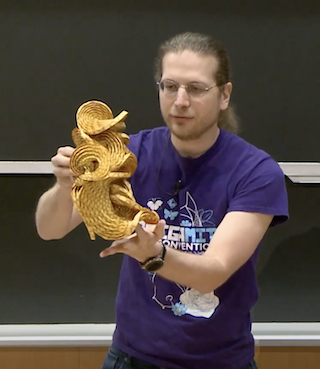6.006 | Spring 2020 | Undergraduate
Introduction to Algorithms
Course Description
This course is an introduction to mathematical modeling of computational problems, as well as common algorithms, algorithmic paradigms, and data structures used to solve these problems. It emphasizes the relationship between algorithms and programming and introduces basic performance measures and analysis techniques …
Course Info
Instructors
Departments
Topics
Learning Resource Types
theaters Lecture Videos
assignment_turned_in Problem Sets with Solutions